Force and Momentum

The net external force acting on an object can be evaluated as the rate of change of momentum. This turns out to be a more fundamental way of stating the force than the use of Newton's second law. Using Newton's second law and momentum:But this limited relationship can be generalized to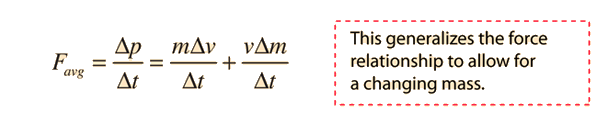and further generalized by calculus methods to include instantaneous rates of change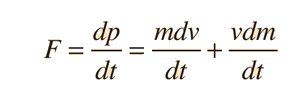This formulation of the force relationship permits varying mass, as in rocket propulsion.

In the language of calculus, we say that the force is defined as the derivative of the momentum. The process above involves the product rule for derivatives.

Index

Limits on Newton's 2nd law

 HyperPhysics***** Mechanics ***** Newton's laws R Nave
Go Back

Rocket Propulsion

The thrust of a rocket can be modeled from a generalization of Newton's 2nd Law to include a variable mass: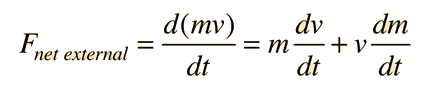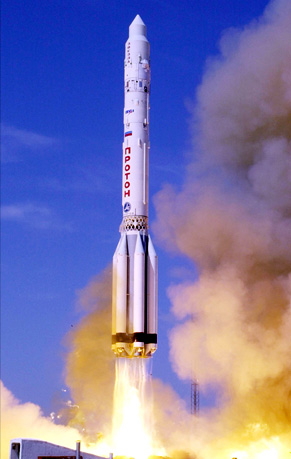In free space this would lead to a rocket velocity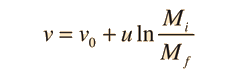Show

and for a rocket launched from the Earth's surface it leads to the expressionShow

at low altitudes where g can be considered to be constant.

(This is the launch of the Zvezda service module of the International Space Station on a Russian Proton-K rocket, July 12, 2000. Image source NASA.)
Index

 HyperPhysics***** Mechanics R Nave
Go Back

Thrust of a Rocket

Rocket thrust results from the high speed ejection of material and does not require any medium to "push against". Conservation of momentum dictates that if material is ejected backward, the forward momentum of the remaining rocket must increase since an isolated system cannot change its net momentum.NASA Space Shuttle launch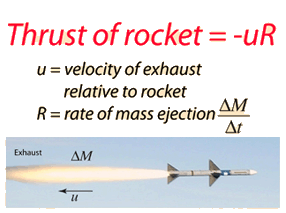Show
Index

Rocket propulsion

 HyperPhysics***** Mechanics R Nave
Go Back

Thrust of a Rocket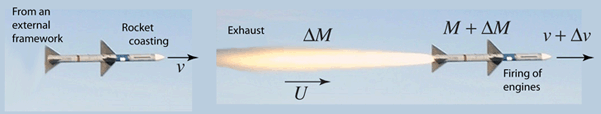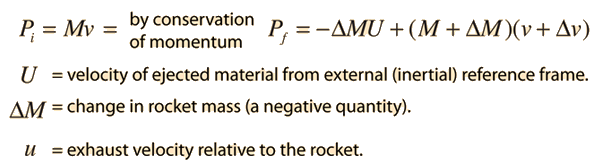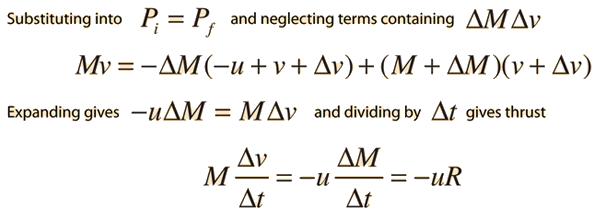Rocket thrust Show differential approach Further discussion of rocket thrust
Index

Rocket propulsion

 HyperPhysics***** Mechanics R Nave
Go Back

Thrust of a Rocket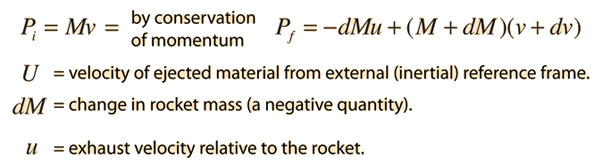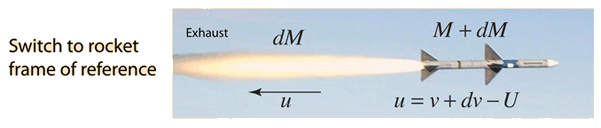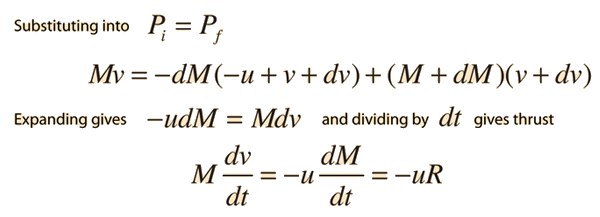Rocket thrust Show algebra approach Further discussion of rocket thrust
Index

Rocket propulsion

 HyperPhysics***** Mechanics R Nave
Go Back

Rocket Thrust Discussion

Developing the expression for rocket thrust involves the application of conservation of momentum in an accelerating reference frame. It can be developed in an approximate fashion using finite differences and algebra. This requires neglecting terms like ΔMΔv. When the appropriate limits are taken to get an instantaneous expression, these approximations disappear since the quantities dM and dt approach zero.
 Show algebra approach Show calculus approach
Index

Rocket propulsion

 HyperPhysics***** Mechanics R Nave
Go Back